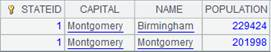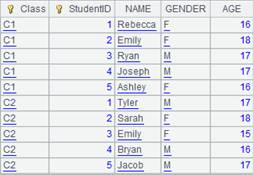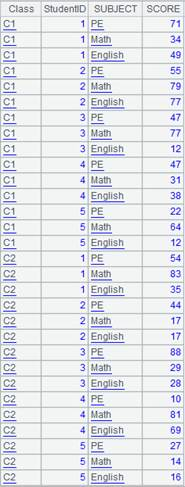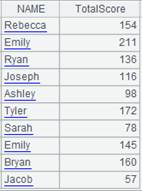# total()

## cs.total()

cs.total(y,…)

 cs 游标 y 聚合函数，仅支持sum/count/max/min/top/avg/iterate，使用iterate(x,a;Gi,…)时不能有参数Gi。

数值/数值序列

 A 1 =demo.cursor("select * from SCORES where CLASS = 'Class one'") 2 =A1.total(sum(SCORE))返回单值 3 =A1.reset() 回转游标移回头 4 =A1.total(sum(SCORE),count(SUBJECT),max(SCORE))按y的顺序返回

## ch.total()

ch.total(y,…)

 ch 管道 y 聚合函数，仅支持sum/count/max/min/top/avg/iterate，使用iterate(x,a;Gi,…)时不能有参数Gi。

 A 1 =demo.cursor("select * from SCORES where CLASS = 'Class one'") 2 =channel() 创建管道 3 =channel() 4 =A1.push(A2,A3) 将游标A1中的数据推送到管道A2、A3， 此时数据不会立即被推送到管道。 5 =A2.total(sum(SCORE)) 对管道A2中的y汇总 6 =A3.total(sum(SCORE),count(SUBJECT),max(SCORE)) 对管道A3中的y…汇总 7 =A1.fetch() 从游标A1中取数，此时才会执行管道中的计算，游标中的数据才真正被推送到管道 8 =A2.result()9 =A3.result()